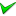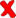# OpenSeaMap-dev:Harbour-layer

The harbour-layer is a clickable layer, which shows harbours, marinas and anchorages from the Harbour-DB(from multiple sources).

## Zoom levels

Actually only OSM-marinas are shown in z=12-18

old
Zahl Zoom → 5 6 7 8 9 10 11 12 13 14 Remarks
124 WPI (large) x x x x x x x x x x klickbar
x x 312 WPI (medium) x x x x x x x x x x klickbar
1175 WPI (small) x x x x x x x x klickbar
2649 WPI (very small) x x x x klickbar
1000 SG (marina) x x klickbar+Link
new
Zahl Zoom → 3 4 5 6 7 8 9 10 11 12 13 14 Remarks
160 WPI (large)x x x x x x x x x x x klickbar
362 WPI (medium)x x x x x x x klickbar
982 WPI (small)x x x x x klickbar
2125 WPI (very small)x x x klickbar
SG (harbour) x x x klickbar+Link
OSM (harbour) x x x
OSM (marina) x x
OSM (anchorage) x
Clustering

In areas with lot of marinas nearby, in middle zoom levels (z=11 to 14) they need to be clustered (depends on zoom level).
In low zoom levels (z=5..7) the importance makes the decision, which harbour is displayed.

## Popup

In a popup we display:

show 2009 2010 201? actual target
Harbour name x xx
Harbour name (WPI LOCODE) x xx
Link to Harbour-Wiki x x xx
Link to Meteogram x x xx
Link to Tide precastx
Link to Wikidata/Wikipediax
Picturex
Link to Wikitravelx